Courses

# Turing Machines And Undecidability Computer Science Engineering (CSE) Notes | EduRev

## Computer Science Engineering (CSE) : Turing Machines And Undecidability Computer Science Engineering (CSE) Notes | EduRev

The document Turing Machines And Undecidability Computer Science Engineering (CSE) Notes | EduRev is a part of the Computer Science Engineering (CSE) Course GATE Computer Science Engineering(CSE) 2022 Mock Test Series.
All you need of Computer Science Engineering (CSE) at this link: Computer Science Engineering (CSE)

## Recursive and Recursive Enumerable Languages

Recursive Enumerable (RE) or Type -0 Language

RE languages or type-0 languages are generated by type-0 grammars. An RE language can be accepted or recognized by Turing machine which means it will enter into final state for the strings of language and may or may not enter into rejecting state for the strings which are not part of the language. It means TM can loop forever for the strings which are not a part of the language. RE languages are also called as Turing recognizable languages.

Recursive Language (REC)

A recursive language (subset of RE) can be decided by Turing machine which means it will enter into final state for the strings of language and rejecting state for the strings which are not part of the language. e.g.; L= {anbncn|n>=1} is recursive because we can construct a turing machine which will move to final state if the string is of the form anbncn else move to non-final state. So the TM will always halt in this case. REC languages are also called as Turing decidable languages. The relationship between RE and REC languages can be shown in Figure 1.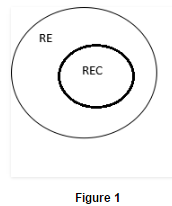Closure Properties of Recursive Languages

• Union: If L1 and If L2 are two recursive languages, their union L1∪L2 will also be recursive because if TM halts for L1 and halts for L2, it will also halt for L1∪L2.
• Concatenation: If L1 and If L2 are two recursive languages, their concatenation L1.L2 will also be recursive. For Example:
` L1= {anbncn|n>=0}  L2= {dmemfm|m>=0} L3= L1.L2 = {anbncndm emfm|m>=0 and n>=0} is also recursive.`
• L1 says n no. of a’s followed by n no. of b’s followed by n no. of c’s. L2 says m no. of d’s followed by m no. of e’s followed by m no. of f’s. Their concatenation first matches no. of a’s, b’s and c’s and then matches no. of d’s, e’s and f’s. So it can be decided by TM.

• Kleene Closure: If L1is recursive, its kleene closure L1* will also be recursive. For Example:
` L1= {anbncn|n>=0} L1*= { anbncn||n>=0}* is also recursive.`

Intersection and complement: If L1 and If L2 are two recursive languages, their intersection L1 ∩ L2 will also be recursive. For Example:

` L1= {anbncndm|n>=0 and m>=0}  L2= {anbncndn|n>=0 and m>=0} L3=L1 ∩ L2 = { anbncndn |n>=0} will be recursive.`

L1 says n no. of a’s followed by n no. of b’s followed by n no. of c’s and then any no. of d’s. L2 says any no. of a’s followed by n no. of b’s followed by n no. of c’s followed by n no. of d’s. Their intersection says n no. of a’s followed by n no. of b’s followed by n no. of c’s followed by n no. of d’s. So it can be decided by turing machine, hence recursive.
Similarly, complementof recursive language L1 which is ∑*-L1, will also be recursive.

Note: As opposed to REC languages, RE languages are not closed under complementon which means complement of RE language need not be RE.

Question 1: Which of the following statements is/are FALSE?

1.For every non-deterministic TM, there exists an equivalent deterministic TM.
2.Turing recognizable languages are closed under union and complementation.
3.Turing decidable languages are closed under intersection and complementation.
4.Turing recognizable languages are closed under union and intersection.

A.1 and 4
B.1 and 3
C.2
D.3

Solution:

Statement 1 is true as we can convert every non-deterministic TM to deterministic TM.
Statement 2 is false as Turing recognizable languages (RE languages) are not closed under complementation.
Statement 3 is true as Turing decidable languages (REC languages) are closed under intersection and complementation.
Statement 4 is true as Turing recognizable languages (RE languages) are closed under union and intersection.

Question 2 : Let L be a language and L’ be its complement. Which one of the following is NOT a viable possibility?

A.Neither L nor L’ is RE.
B.One of L and L’ is RE but not recursive; the other is not RE.
C.Both L and L’ are RE but not recursive.
D.Both L and L’ are recursive.

Solution:

Option A is correct because if L is not RE, its complementation will not be RE. Option B is correct because if L is RE, L’ need not be RE or vice versa because RE languages are not closed under complementation.
Option C is false because if L is RE, L’ will not be RE. But if L is recursive, L’ will also be recursive and both will be RE as well because REC languages are subset of RE. As they have mentioned not to be REC, so option is false.
Option D is correct because if L is recursive L’ will also be recursive.

Question 3: Let L1 be a recursive language, and let L2 be a recursively enumerable but not a recursive language. Which one of the following is TRUE?

A.L1′ is recursive and L2′ is recursively enumerable
B.L1′ is recursive and L2′ is not recursively enumerable
C.L1′ and L2′ are recursively enumerable
D.L1′ is recursively enumerable and L2′ is recursive

Solution:

Option A is False as L2’ can’t be recursive enumerable (L2 is RE and RE are not closed under complementation).
Option B is correct as L1’ is REC (REC languages are closed under complementation) and L2’ is not recursive enumerable (RE languages are not closed under complementation).
Option C is False as L2’ can’t be recursive enumerable (L2 is RE and RE are not closed under complementation).
Option D is False as L2’ can’t be recursive enumerable (L2 is RE and RE languages are not closed under complementation). As REC languages are subset of RE, L2’ can’t be REC as well.

## Turing Machine

Turing Machine was invented by Alan Turing in 1936 and it is used to accept Recursive Enumerable Languages (generated by Type-0 Grammar).

A turing machine consists of a tape of infinite length on which read and writes operation can be performed. The tape consists of infinite cells on which each cell either contains input symbol or
a special symbol called blank. It also consists of a head pointer which points to cell currently being read and it can move in both directions. A TM is expressed as a 7-tuple (Q, T, B, ∑, δ, q0, B, F) where:

• Q is a finite set of states
• T is the tape alphabet (symbols which can be written on Tape)
• B is blank symbol (every cell is filled with B except input alphabet initially)
• is the input alphabet (symbols which are part of input alphabet)
• δ is a transition function which maps Q × T → Q × T × {L,R}. Depending on its present state and present tape alphabet (pointed by head pointer), it will move to new state, change the tape symbol (may or may not) and move head pointer to either left or right.
• q0 is the initial state
• F is the set of final states. If any state of F is reached, input string is accepted.

Let us construct a turing machine for L={0n1n|n>=1}

• Q = {q0,q1,q2,q3} where q0 is initial state.
• T = {0,1,X,Y,B} where B represents blank.
• ∑ = {0,1}
• F = {q3}

Transition function δ is given in Table 1 as: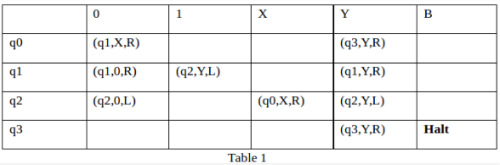Illustration

Let us see how this turing machine works for 0011. Initially head points to 0 which is underlined and state is q0 as: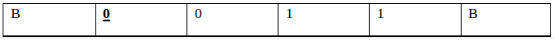The move will be δ(q0, 0) = (q1, X, R). It means, it will go to state q1, replace 0 by X and head will move to right as: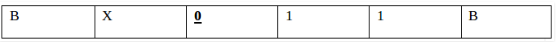The move will be δ(q1, 0) = (q1, 0, R) which means it will remain in same state and without changing any symbol, it will move to right as: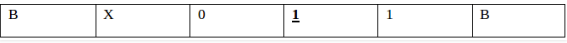The move will be δ(q1, 1) = (q2, Y, L) which means it will move to q2 state and changing 1 to Y, it will move to left as: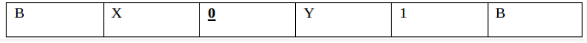Working on it in the same way, the machine will reach state q3 and head will point to B as shown: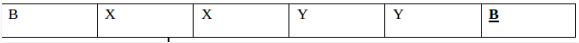Using move δ(q3, B) = halt, it will stop and accepted.

Note:

• In non-deterministic turing machine, there can be more than one possible move for a given state and tape symbol, but non-deterministic TM does not add any power.
• Every non-deterministic TM can be converted into deterministic TM.
• In multi-tape turing machine, there can be more than one tape and corresponding head pointers, but it does not add any power to turing machine.
• Every multi-tape TM can be converted into single tape TM.

Question: A single tape Turing Machine M has two states q0 and q1, of which q0 is the starting state. The tape alphabet of M is {0, 1, B} and its input alphabet is {0, 1}. The symbol B is the blank symbol used to indicate end of an input string. The transition function of M is described in the following table.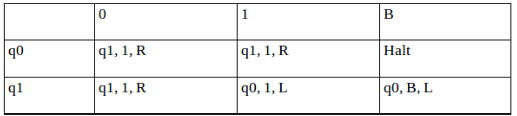The table is interpreted as illustrated below. The entry (q1, 1, R) in row q0 and column 1 signifies that if M is in state q0 and reads 1 on the current tape square, then it writes 1 on the same tape square, moves its tape head one position to the right and transitions to state q1. Which of the following statements is true about M?

1. M does not halt on any string in (0 + 1)+
2. M does not halt on any string in (00 + 1)*
3. M halts on all string ending in a 0
4. M halts on all string ending in a 1

Solution:  Let us see whether machine halts on string ‘1’. Initially state will be q0, head will point to 1 as: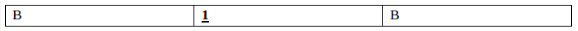Using δ(q0, 1) = (q1, 1, R), it will move to state q1 and head will move to right as: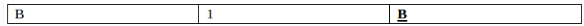Using δ(q1, B) = (q0, B, L), it will move to state q0 and head will move to left as: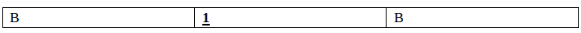It will run in the same way again and again and not halt.

Option D says M halts on all string ending with 1, but it is not halting for 1. So, option D is incorrect.

Let us see whether machine halts on string ‘0’. Initially state will be q0, head will point to 1 as: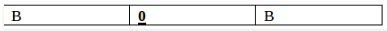Using δ(q0, 0) = (q1, 1, R), it will move to state q1 and head will move to right as: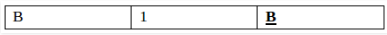Using δ(q1,B)=(q0,B,L), it will move to state q0 and head will move to left as: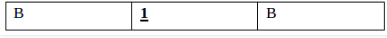It will run in the same way again and again and not halt.

Option C says M halts on all string ending with 0, but it is not halting for 0. So, option C is incorrect.

Option B says that TM does not halt for any string (00 + 1)*. But NULL string is a part of (00 + 1)* and TM will halt for NULL string. For NULL string, tape will be,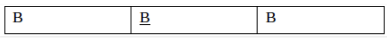Using δ(q0, B) = halt, TM will halt. As TM is halting for NULL, this option is also incorrect.
So, option (A) is correct.

## Undecidability and Reducibility

Decidable Problems

A problem is decidable if we can construct a Turing machine which will halt in finite amount of time for every input and give answer as ‘yes’ or ‘no’. A decidable problem has an algorithm to determine the answer for a given input.

Examples

• Equivalence of two regular languages: Given two regular languages, there is an algorithm and Turing machine to decide whether two regular languages are equal or not.
• Finiteness of regular language: Given a regular language, there is an algorithm and Turing machine to decide whether regular language is finite or not.
• Emptiness of context free language: Given a context free language, there is an algorithm whether CFL is empty or not.

Undecidable Problems

A problem is undecidable if there is no Turing machine which will always halt in finite amount of time to give answer as ‘yes’ or ‘no’. An undecidable problem has no algorithm to determine the answer for a given input.

Examples

• Ambiguity of context-free languages: Given a context-free language, there is no Turing machine which will always halt in finite amount of time and give answer whether language is ambiguous or not.
• Equivalence of two context-free languages: Given two context-free languages, there is no Turing machine which will always halt in finite amount of time and give answer whether two context free languages are equal or not.
• Everything or completeness of CFG: Given a CFG and input alphabet, whether CFG will generate all possible strings of input alphabet (∑*)is undecidable.
• Regularity of CFL, CSL, REC and REC: Given a CFL, CSL, REC or REC, determining whether this language is regular is undecidable.

Note: Two popular undecidable problems are halting problem of TM and PCP (Post Correspondence Problem). Semi-decidable Problems
A semi-decidable problem is subset of undecidable problems for which Turing machine will always halt in finite amount of time for answer as ‘yes’ and may or may not halt for answer as ‘no’.
Relationship between semi-decidable and decidable problem has been shown in Figure 1 as: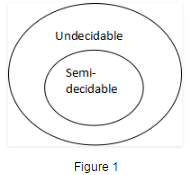Rice’s Theorem

Every non-trivial (answer is not known) problem on Recursive Enumerable languages is undecidable.e.g.; If a language is Recursive Enumerable, its complement will be recursive enumerable or not is undecidable.

Reducibility and Undecidability

Language A is reducible to language B (represented as A≤B) if there exists a function f which will convert strings in A to strings in B as:

`w ɛ A <=> f(w) ɛ B`

Theorem 1: If A≤B and B is decidable then A is also decidable.
Theorem 2: If A≤B and A is undecidable then B is also undecidable.

Question: Which of the following is/are undecidable?

1.  G is a CFG. Is L(G)=ɸ?
2.  G is a CFG. Is L(G)=∑*?
3. M is a Turing machine. Is L(M) regular?
4.  A is a DFA and N is an NFA. Is L(A)=L(N)?

A. 3 only
B. 3 and 4 only
C. 1, 2 and 3 only
D. 2 and 3 only

Explanation:

• Option 1 is whether a CFG is empty or not, this problem is decidable.
• Option 2 is whether a CFG will generate all possible strings (everything or completeness of CFG), this problem is undecidable.
• Option 3 is whether language generated by TM is regular is undecidable.
• Option 4 is whether language generated by DFA and NFA are same is decidable. So option D is correct.

Question: Which of the following problems are decidable?

1.  Does a given program ever produce an output?
2.  If L is context free language then L’ is also context free?
3.  If L is regular language then L’ is also regular?
4.  If L is recursive language then L’ is also recursive?

A. 1,2,3,4
B. 1,2
C. 2,3,4
D. 3,4

Explanation:

• As regular and recursive languages are closed under complementation, option 3 and 4 are decidable problems.
• Context free languages are not closed under complementation, option 3 is undecidable.
• Option 1 is also undecidable as there is no TM to determine whether a given program will produce an output. So, option D is correct.

Question: Consider three decision problems P1, P2 and P3. It is known that P1 is decidable and P2 is undecidable. Which one of the following is TRUE?

A. P3 is undecidable if P2 is reducible to P3
B. P3 is decidable if P3 is reducible to P2’s complement
C. P3 is undecidable if P3 is reducible to P2
D. P3 is decidable if P1 is reducible to P3
Explanation:

• Option A says P2≤P3. According to theorem 2 discussed, if P2 is undecidable then P3 is undecidable. It is given that P2 is undecidable, so P3 will also be undecidable. So option (A) is correct.
• Option C says P3≤P2. According to theorem 2 discussed, if P3 is undecidable then P2 is undecidable. But it is not given in question about undecidability of P3. So option (C) is not correct.
• Option D says P1≤P3. According to theorem 1 discussed, if P3 is decidable then P1 is also decidable. But it is not given in question about decidability of P3. So option (D) is not correct.
• Option (B) says P3≤P2’. According to theorem 2 discussed, if P3 is undecidable then P2’ is undecidable. But it is not given in question about undecidability of P3. So option (B) is not correct.

Offer running on EduRev: Apply code STAYHOME200 to get INR 200 off on our premium plan EduRev Infinity!

## GATE Computer Science Engineering(CSE) 2022 Mock Test Series

131 docs|167 tests

,

,

,

,

,

,

,

,

,

,

,

,

,

,

,

,

,

,

,

,

,

;